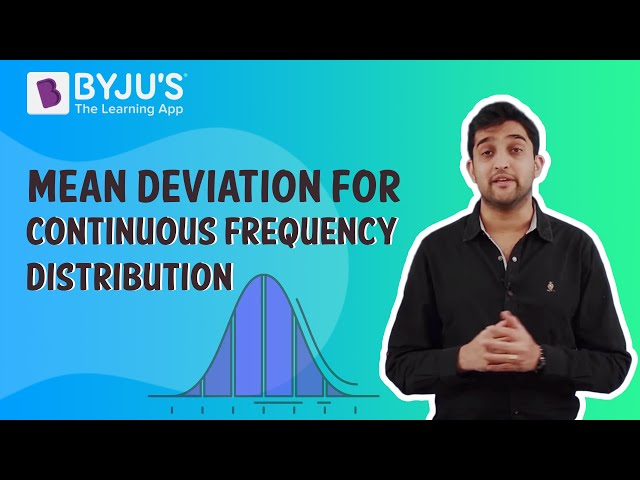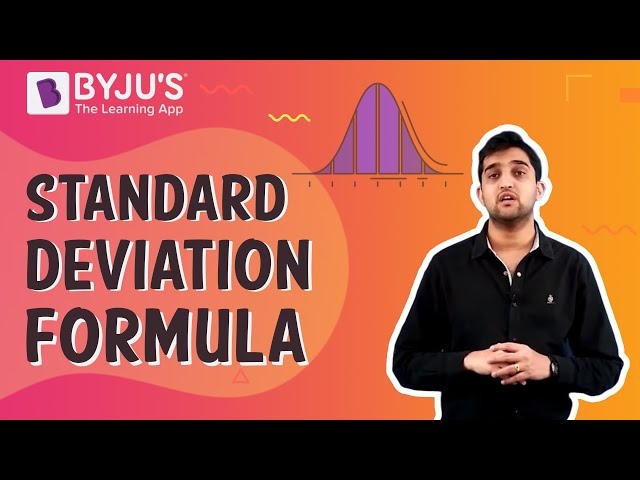# Statistics Class 11

## Statistics Class 11 Notes

In statistics class 11, the importance of statistics in studying the measures of dispersion and the methods of calculating the grouped and ungrouped data has been explained. In applied mathematics, statistics is a branch that deals with the collection, organization and interpretation of data. It is similar to the study of the probability of events occurring based on the collection of data or the known quantities of data. Some of the concepts with examples and the scope of the statistics are explained in the Class 11 maths chapter 15 Statistics.

## Statistics Class 11 Chapter 15 Concepts

The concepts covered in this chapter 15 of Class 11 Statistics are:

• Introduction
• Measures of Dispersion
• Range
• Mean Deviation
• Variance and Standard Deviation
• Analysis of Frequency Distributions

Let’s have a look at the brief explanation of all these concepts with examples given below:

A measure of central tendency gives us a rough idea of wherever data points are centred. However, to better understand the data, we should know how the data are scattered or how much they are bunched around a measure of central tendency. However, the measures of central tendency are not adequate to give exhaustive information about a given data. Variability is another essential factor that is expected to be studied in statistics. Similar to the measures of central tendency, we need to have a unique number that describes the variability, and this single number is called a measure of dispersion. In this chapter, you will learn some of the important measures of dispersion and the methods for calculating these measured for both ungrouped and grouped data.

## Measures of Dispersion

The dispersion or scatter in the data is measured based on the observations and the types of the measure of central tendency. The different types of measures of dispersion are:

• Range
• Quartile deviation
• Mean deviation
• Standard deviation.

In Class 11 statistics, you get an explicit knowledge of all the measures of dispersion except quartile deviation, which will be studied in your higher classes.

## Range

The range is the difference between the maximum value and the minimum value

Range = Maximum Value – Minimum Value

## Mean Deviation

Mean deviation is the basic measure of deviations from value, and the value is generally a mean value or a median value. In order to find out the mean deviation, first take the mean of deviation for the observations from value is d = x – a Here x is the observation, and a is the fixed value.

The basic formula to find out the mean deviation is

Mean Deviation = Sum of absolute values of deviations from ‘a’ / Number of observations

### Mean Deviation for Ungrouped Data

Calculation of mean deviation for ungrouped data involves the following steps :

Let us assume the observations x1, x2, x3, …..xn

Step 1: Calculate the central tendency measures to find the mean deviation and let it be ‘a’.

Step 2: Find the deviation of xi from a, i.e., x1 – a, x2– a, x3 – a,. . . , xn– a

Step 3: Find the absolute values of the deviations, i.e., | x1 – a |, | x2– a |, |x3 – a|,. . . ,|xn– a| and drop the minus sign (–), if it is there,

Step 4: calculate the mean of the absolute values of the deviations. This mean obtained is the mean deviation about a, i.e.,

$$\begin{array}{l}M.D (a)= \frac{\sum_{i=1}^{n}\left |x_{i}-a \right |}{n}\end{array}$$

$$\begin{array}{l}M.D (\bar{x})= \frac{\sum_{i=1}^{n}\left |x_{i}-\bar{x} \right |}{n}\text{, where } \bar{x}\text{ = Mean}\end{array}$$

$$\begin{array}{l}M.D (M)= \frac{\sum_{i=1}^{n}\left |x_{i}-M \right |}{n}\end{array}$$

Where M = Median

### Mean Deviation for Grouped Data

The data can be grouped into two ways namely,

• Discrete frequency distribution
• Continuous frequency distribution

The methods of finding the mean deviation for both the types are given below

## Discrete Frequency Distribution

Here, the given data consist of n distinct values x1, x2, x3,….xn has frequencies f1, f2, f3,….fn respectively. This data is represented in the tabular form as and is called discrete frequency distribution, and the data are given below

 x x1 x2 x3 …… xn f f1 f2 f3 …… fn

$$\begin{array}{l}\text{First find the mean } \bar{x} \text{ , using the given formula:}\end{array}$$
$$\begin{array}{l}\bar{x}=\frac{\sum_{i=1}^{n}x_{i}f_{i}}{\sum_{i=1}^{n}f_{i}}=\frac{1}{N}\sum_{i=1}^{n}x_{i}f_{i}\end{array}$$

Where the numerator denotes the sum of products of observations xi with the respective frequencies fi and the denominator denotes the sum of frequencies.

Now take the absolute values,

$$\begin{array}{l}\left | x_{i}-\bar{x} \right |\text{ , for all i = 1, 2, 3, ..n}\end{array}$$

Therefore, the required mean deviation about the mean is given by

$$\begin{array}{l}M.D(\bar{x})=\frac{\sum_{i=1}^{n}f_{i}\left | x_{i}-\bar{x} \right |}{\sum_{i=1}^{n}f_{i}}=\frac{1}{N}\sum_{i=1}^{n}f_{i}\left | x_{i}-\bar{x} \right |\end{array}$$

To find the mean deviation about the median for the given discrete frequency distribution. First arrange the observation in ascending order to get cumulative frequency which is equal to or greater than N/2, where N is the sum of the frequencies.

Therefore, the mean deviation for median is given by,

$$\begin{array}{l}M.D(M)= \frac{1}{N}\sum_{i=1}^{n}f_{i}\left | x_{i}-M \right |\end{array}$$

Also, check:

## Continuous Frequency Distribution

To find the mean deviation for the continuous frequency distribution, assume that the frequency in each class is centred at its midpoint. After finding the midpoint, proceed further to find the mean deviation similar to the discrete frequency distribution.

### Standard Deviation

The positive square root of the variance is called standard deviation (S.D) and it is denoted by the symbol, 𝝈 and the formula to find S.D is given by

$$\begin{array}{l}\sigma =\sqrt{\frac{1}{n}\sum_{i=1}^{n}(x_{i}-\bar{x})^{2}}\end{array}$$

Where the variance is denoted as 𝝈2 and it is given by

$$\begin{array}{l}\sigma^{2} =\frac{1}{n}\sum_{i=1}^{n}(x_{i}-\bar{x})^{2}\end{array}$$

To understand more about standard deviation, visit here.

### Mean Deviation### Standard Deviation### Class 11 Statistics Examples

Question 1: Find the range and mean deviation about the mean for the following data:

6, 7, 10, 12, 13, 4, 8, 12

Solution:

Given data: 6, 7, 10, 12, 13, 4, 8, 12

Range = Maximum value – Minimum value

= 13 – 4
= 9

Now, we need to calculate the mean.

Mean (x̄) = (6 + 7 + 10 + 12 + 13 + 4 + 8 + 12)/8 = 72/8 = 9

Let us calculate the deviations, i.e. xi – x̄

6 – 9, 7 – 9, 10 – 9, 12 – 9, 13 – 9, 4 – 9, 8 – 9, 12 – 9,

or

–3, –2, 1, 3, 4, –5, –1, 3

The absolute deviations are:

3, 2, 1, 3, 4, 5, 1, 3

As we know, the formula of mean deviation about the mean is

$$\begin{array}{l}M.D (\bar{x})= \frac{\sum_{i=1}^{n}\left |x_{i}-\bar{x} \right |}{n}\text{, where } \bar{x} \text{= Mean}\end{array}$$

So, M.D(x̄) = (3 + 2 + 1 + 3 + 4 + 5 + 1 + 3)/8 = 22/8 = 2. 75

Therefore,

Range = 9

Mean deviation about the mean = 2.75

Question 2:

Obtain the variance and standard deviation for the following data :

 xi 4 8 11 17 20 24 32 fi 3 5 9 5 4 3 1

Solution :

Data in tabular form :

 xi fi fi xi $$\begin{array}{l}x_{i}-\bar{x}\end{array}$$ $$\begin{array}{l}(x_{i}-\bar{x})^{2}\end{array}$$ $$\begin{array}{l}f_{i}(x_{i}-\bar{x})^{2}\end{array}$$ 4 8 11 17 20 24 32 3 5 9 5 4 3 1 12 40 99 85 80 72 32 -10 -6 -3 3 6 10 18 100 38 9 9 36 100 324 300 180 81 45 144 300 324 30 420 1374

Here, N = 30 = fi

$$\begin{array}{l}\sum_{i=1}^{7}f_{i}x_{i}=420\end{array}$$
$$\begin{array}{l}\sum_{i=1}^{7}f_{i}(x_{i}-\bar{x})^{2}=1374\end{array}$$

Therefore,

$$\begin{array}{l}\bar{x}=\frac{\sum_{i=1}^{7}f_{i}x_{i}}{N}\end{array}$$

= 420/30

= 14

$$\begin{array}{l}\bar{x}= 14\end{array}$$
$$\begin{array}{l}Variance = \sigma^{2} =\frac{1}{n}\sum_{i=1}^{n}(x_{i}-\bar{x})^{2}\end{array}$$
$$\begin{array}{l}\sigma^{2} =\frac{1}{n}\sum_{i=1}^{7}f_{i}(x_{i}-\bar{x})^{2}\end{array}$$

= 1374/30 = 45.8

Variance = 45.8

$$\begin{array}{l}\text{Standard Deviation, } \sigma =\sqrt{45.8}= 6.77\end{array}$$

Register with BYJU’S learning app to get more information on Maths class 11 chapters and other related articles and start practice with the problems.